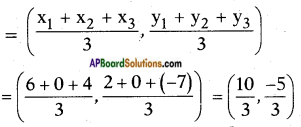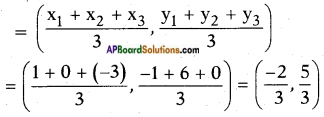AP State Board Syllabus AP SSC 10th Class Maths Textbook Solutions Chapter 7 Coordinate Geometry Ex 7.2 Textbook Questions and Answers.

## AP State Syllabus SSC 10th Class Maths Solutions 7th Lesson Coordinate Geometry Exercise 7.2

### 10th Class Maths 7th Lesson Coordinate Geometry Ex 7.2 Textbook Questions and Answers

Question 1.
Find the coordinates of the point which divides the line segment joining the points (-1, 7) and (4, -3) in the ratio 2 :3.
Given points P (-1, 7) and Q (4, – 3). Let ‘R’ be the required point which divides $$\overline{\mathrm{PQ}}$$ in the ratio 2:3. Then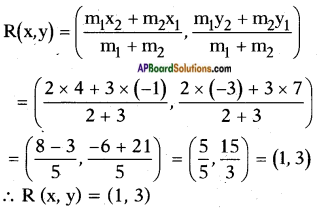Question 2.
Find the coordinates of the points of trisection of the line segment joining (4, -1) and (-2, -3).
Given points A (4, – 1) and B (- 2, – 3) Let P and Q be the points of trisection
of $$\overline{\mathrm{AB}}$$, then AP = PQ = QB.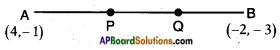∴ P divides $$\overline{\mathrm{AB}}$$ internally in the ratio 1 : 2.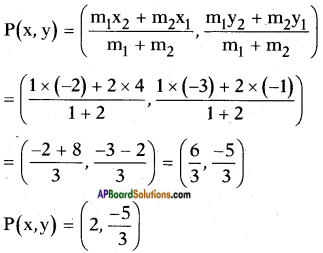Also, Q divides $$\overline{\mathrm{AB}}$$ in the ratio 2 : 1 internally.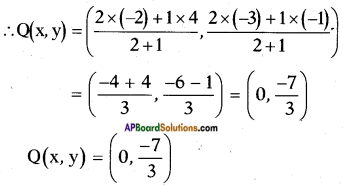Question 3.
Find the ratio in which the line segment joining the points (-3, 10) and (6, -8) is divided by (-1, 6).
Let the point (-1, 6) divides the line segment joining the points (-3, 10) and (6, -8) in a ratio of m : n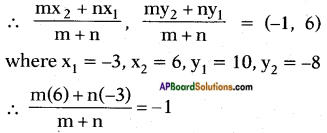⇒ 6m – 3n = -(m + n) = -m – n
⇒ 6m + m = – n + 3n
⇒ 7m = 2n
⇒ $$\frac{m}{n}=\frac{2}{7}$$
⇒ m : n = 2 : 7
∴ The point (-1, 6) divides the given line segment in a ratio of 2 : 7.Question 4.
If (1, 2), (4, y), (x, 6) and (3, 5) are the vertices of a parallelogram taken in order, find x and y.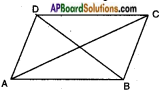Given: ▱ ABCD is a parallelogram where A (1, 2), B (4, y), C (x, 6) and D (3, 5).
In a parallelogram, diagonals bisect each other.
i.e., the midpoints of the diagonals coincide with each other.
i.e.,midpoint of AC = midpoint of BD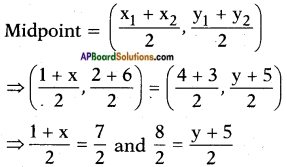⇒ 1 + x = 7 and 8 = y + 5
⇒ x = 7 – 1 and y = 8 – 5
∴ x = 6 and y = 3.

Question 5.
Find the coordinates of a point A, where AB is the diameter of a circle whose centre is (2, -3) and B is (1, 4).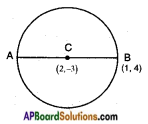Given:
A circle with centre ‘C’ (2, -3). $$\overline{\mathrm{AB}}$$ is a diameter where
B = (1, 4); A = (x, y).
C is the midpoint of AB.
[∵ Centre of a circle is the midpoint of the diameter]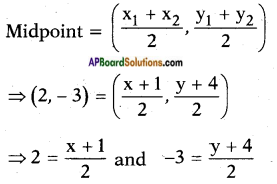4 = x + 1 and – 6 = y + 4
⇒ x = 4 – 1 = 3 and y = -6 – 4 = -10
A (x, y) = (3, -10)Question 6.
If A and B are (-2, -2) and (2, -4) respectively. Find the coordinates of P such that AP = $$\frac{3}{7}$$ AB and P lies on the segment AB.
Given: A (- 2, – 2) and B (2, – 4)
P lies on AB such that AP = latex]\frac{3}{7}[/latex] AB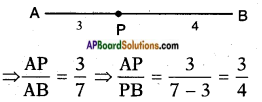i.e., P divides $$\overline{\mathrm{AB}}$$ in the ratio 3 : 4 By section formula,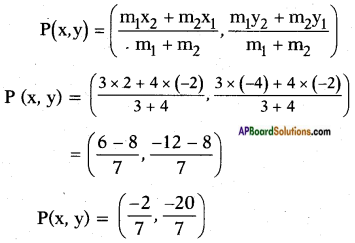Question 7.
Find the coordinates of points which divide the line segment joining A (-4, 0) and B (0, 6) into four equal parts.
Given, A (- 4, 0) and B (0, 6).
Let P, Q and R be the points which divide AB into four equal parts.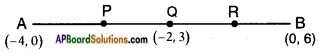P divides $$\overline{\mathrm{AB}}$$ in the ratio 1 : 3, Q → 1 : 1 and R → 3 : 1 Use section formula to find P, Q and R.
Then, Q is the midpoint of $$\overline{\mathrm{AB}}$$
P is the midpoint of $$\overline{\mathrm{AQ}}$$
R is the midpoint of $$\overline{\mathrm{QB}}$$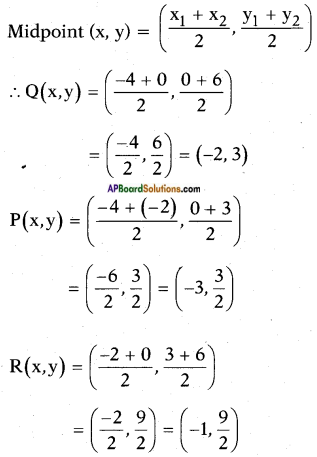Question 8.
Find the coordinates of the points which divides the line segment joining A(-2, 2) and B(2, 8) into four equal parts.
Given, A (- 2, 2) and B (2, 8).
Let P, Q and R be the points which divide AB into four equal parts.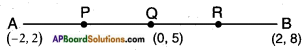Then, Q is the midpoint of $$\overline{\mathrm{AB}}$$
P is the midpoint of $$\overline{\mathrm{AQ}}$$
R is the midpoint of $$\overline{\mathrm{QB}}$$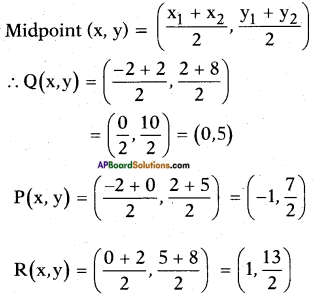Question 9.
Find the coordinates of the point which divide the line segment joining the points (a + b, a-b) and (a-b, a + b) in the ratio 3 : 2 internally.
Given : A (a + b, a – b) and B (a – b, a + b).
Let P (x, y) divides $$\overline{\mathrm{AB}}$$ in the ratio 3 : 2 internally.
Section formula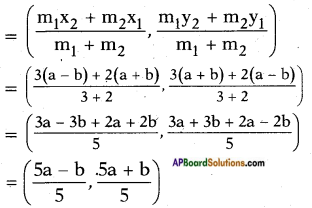Question 10.
Find the coordinates of centroid of the triangle with following vertices:
i) (-1, 3), (6, -3) and (-3, 6)
Given: △ABC in which- A (- 1, 3), B (6, -3) and C (-3, 6)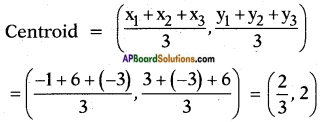ii) (6, 2), (0, 0) and (4, -7)